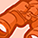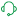# New to Qlik Sense

If you’re new to Qlik Sense, start with this Discussion Board and get up-to-speed quickly.

cancel
Showing results for
Did you mean:Contributor II

## How to add second condition?

Hi Eveyone,

I have a code as below:

``````if(([BP2]='XXX'),
Sum(
RangeMax(
([# STI Plan] * (([Cprice] - ([XXX CDF] / 1.23 / [ER Plan] * (1 - T2_GP) * (1 - T1_GP))))),0)),
Sum(
RangeMax(
([# STI Plan] * (([Cprice] - ([CDF Calc] / 1.23 / [ER Plan] * (1 - T2_GP) * (1 - T1_GP))))),0)))
``````

How to add second condition? Else_expr as you can see in both cases is the same.

``````if(((Front='1'),
Sum(
RangeMax(
([# STI Plan] * (([Cprice] - ([SRP] / 1.23 / [ER Plan] * (1 - T2_GP) * (1 - T1_GP))))),0)),
Sum(
RangeMax(
([# STI Plan] * (([Cprice] - ([CDF Calc] / 1.23 / [ER Plan] * (1 - T2_GP) * (1 - T1_GP))))),0)))
``````

Labels (2)

• ### Filter